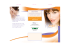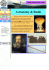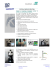# TSBB09 Computer Exercise A The Digital Camera Developed by Per-Erik Forss´

## Transcription

TSBB09 Computer Exercise A The Digital Camera Developed by Per-Erik Forss´
```TSBB09 Computer Exercise A
The Digital Camera
Developed by Per-Erik Forss´en and Maria Magnusson 2008.
Computer Vision Laboratory, Link¨oping University, Sweden
Last update: Maria Magnusson, Klas Nordberg and Marcus Wallenberg,
November 2014
Contents
1 Preliminaries
1.1 Displaying images in Matlab . . . . . . . . . . . . . . . . . .
2.1 Shading correction . . . . .
2.2 Deinterlacing . . . . . . . .
2.3 Bayer pattern interpolation
2.4 Noise measurements . . . .
.
.
.
.
1
.
.
.
.
.
.
.
.
.
.
.
.
.
.
.
.
.
.
.
.
.
.
.
.
.
.
.
.
.
.
.
.
.
.
.
.
.
.
.
.
.
.
.
.
.
.
.
.
.
.
.
.
.
.
.
.
.
.
.
.
.
.
.
.
.
.
.
.
.
.
.
.
1
2
3
3
9
11
13
18
Preliminaries
Before attending the computer exercise it is necessary to read through the
course material  as well as this guide to the exercise. The guide contains
a few home exercises to be answered before the session. They are all clearly
marked with a pointing finger.
Extra
Implementation exercises marked with an “Extra” box may be temporarily
skipped and completed when the other exercises are finished.
Examples of Matlab commands frequently used are imread, imshow, size,
zeros, and reshape. If you are unfamiliar with any of these commands, you
should use the Matlab help function to find out how what they do.
1
1.1
Displaying images in Matlab
There are two commands for displaying images in Matlab, imagesc and
imshow. The second one automatically scales the image so that the pixels
become rectangular, removes the axes and sets the colortable to grayscale.
Consequently
imagesc(Im);
axis image;
axis off;
colormap gray;
or
imshow(Im, []);
give the same result. In the commands above, Im contains the image and []
automatically finds the minimum and maximum of the image and displays
it with a linear scale in between. Note that [] is not needed in imagesc.
There exists also other colortables, i.e. jet, or it is possible to design your
own colortable.
If another range of pixel values is desired, you can write
imagesc(Im, [min max]);
or
imshow(Im, [min max]);
giving that the image is displayed with a linear scale between the values
min and max.
The command
colorbar;
is useful in connection with displaying images. It shows how the colors
in the image correspond to pixel values.
The command
imshow(Im);
display the image with a linear scale between 0 and 1. Consequently, there
is no automatical scaling due to pixel values. When true color images (with
three colorplanes R ,G, and B) are going to be displayed, the pixel values
should be scaled to the interval between 0 and 1.
One exception to what have been said above is 8-bit images of class uint8.
For such images just
imshow(Im);
works fine.
2
2
Today digital cameras are ubiquitous. Almost all consumer still image cameras use digital sensors, and digital cameras can also be found in most mobile
phones and laptops. In this assignment we will look at the output from a
sensor that uses a Bayer pattern colour filter array (CFA) to capture colour
images. This is the most common CFA in use today, and it can be found
in both still-image cameras and camcorders. We will also have a look at
interlaced images, which are common on analogue video camcorders. These
images have been digitized with a framegrabber.
The different tasks of this exercise are relatively independent of each other
and, therefore, they can be solved in arbitrary order. Some useful files are
located in a common directory. Start by adding a path to these directories
in the Matlab window:
2.1
Shading correction is described in the appendix. Our goal in this assignment
is to correct for uneven illumination. Note that uneven illumination is only
can also be applied to correct errors inside the camera caused by the sensor
elements and the optics. A main application in consumer cameras is to
correct for vignetting. This procedure is rather called vignetting correction
Home exercise Assume that you have two reference images, one dark
and one bright, given by bA (x, y) and bB (x, y), respectively. Also assume
that you have an original image b(x, y) taken with the camera. A corrected
image will be computed as fˆ(x, y) = c(x, y) (b(x, y) + d(x, y)). The values in
bA (x, y) should be corrected to fˆA (x, y) and the values in bB (x, y) should be
corrected to fˆB (x, y). Note that fˆA (x, y) and fˆB (x, y) are constant values
independent of (x, y).
QUESTION: Express c and d as functions of bA , bB , fˆA and fˆB .
3
Next we will perform shading correction in practice. The following procedure
• A black cloth was selected as background. Uneven illumination was
introduced by tilting the lamp. The introduced uneven illumination
was not changed during the rest of the experiment. A network camera
was arranged to “look at” the scene.
• Then, the background was covered with a white cloth to serve as the
bright reference image. The bright reference image bB (x, y) was taken
and named whiteimage.
• Then, the white cloth was removed so that the black cloth became
visible again. The first dark reference image bB (x, y) was taken and
named darkimage. This dark reference image is dark gray rather than
black, because the scene was highly illuminated by the lamp.
• Then, the lens was blocked and a second dark reference image bB (x, y)
was taken and named blackimage. This image is almost black.
• Then, the blocking of the lens was removed and a scene with different
objects was arranged on the black cloth background. The original
image b(x, y) was taken and named origimage.
it. The four images mentioned above should show up. We now have three
reference images. The second dark reference image produced by blocking of
the lens is the most common type of dark reference image.
QUESTION: In our case, however, it may be a little better to instead use
the dark gray image as the dark reference image – why? Use the figure
The shading corrected image should not have intensity values that deviate
too much from the original image. Therefore, the reference levels should be
chosen wisely. The value fˆB can, for example, be selected to the average of
the bright image and the value fˆA can be selected to the average of the dark
reference image: mean(mean(darkimage))
QUESTION: Which values do you select for fˆA and fˆB ?
4
b(x,y)
f(x,y), f(x,y)
black
dark
gray
bright
Write Matlab code for shading correction and be prepared to show it later
to the teacher. It can be helpful to use the jet colormap during development
to see better, i.e. colormap(jet).
QUESTION: Did you succeed in correcting for the uneven illumination?
QUESTION: What is the result if you try the black image as a dark reference
image? Which values do you select for fˆA and fˆB ?
QUESTION: Is your corrected image noisier than the original image?
QUESTION: In practice, the bright and dark reference images are often
obtained by averaging a number of bright and dark images, respectively.
What could be the reason for this?
5
2.2
Deinterlacing
Analogue video comes in two main formats: interlaced and progressive scan.
In progressive scan each image is taken at one point in time, alternatively
the lines are taken sequentially one at the time from top to bottom. For
interlaced scans, however, the odd scan lines come from one point in time,
and the even scan lines come from the next following time point. These two
sets of scan lines are called the fields of the interlaced image. Interlaced mode
allows double the temporal frequency at the same bandwidth as progressive
mode, which is an advantage in the old systems for broadcast television.
The disadvantage is that still images from an interlaced sequence typically
contain even and odd lines from two different time points, making such an
image appear distorted if there is much motion in the image.
When analogue video is digitized, using a framegrabber, the images obtained
also have interlaced scan lines. We will now look at such an image.
Start Matlab and add paths as described in the beginning of the Task
image into the variable im, and make sure you convert it to double format
using the function im2double. (This function also scales the image to the
interval between 0 and 1.) Now look at the image using imshow. Use the
zoom tool to examine details.
QUESTION: What is moving, and what is stationary in this image? What
is the direction of the motion?
Next, generate two masks, one for each of the two interlaced fields:
[rows,cols,ndim]=size(im);
We can use these to mask out the individual fields in the image as follows:
6
QUESTION: Is the motion easier to see now?
The two images we just created have every second line set to zero. You
should now fill in the missing values using interpolation. You may use the
convolution command conv2 and the interpolation kernel: f=[1 2 1]’/2;.
QUESTION: Why is 2 (and not 4) a good normalization factor here?
Use the following piece of code to create the two images im1 and im2. Two
of the lines below are incomplete, and you are expected to add the proper
calls to conv2.
im1 = zeros(size(im));
im2 = zeros(size(im));
for k=1:3,
bk=im(:,:,k);
im1(:,:,k)= ... insert your command here...
im2(:,:,k)= ... insert your command here...
end
QUESTION: What are the missing commands?
QUESTION: Look at the result with imshow. Can you find any artifacts of
7
2.3
Bayer pattern interpolation
Start Matlab and add paths as described in the beginning of the Task
section. Load one of the images from the folder bayer/ into the variable im.
Also make sure you convert the image to double format using the function
im2double. (This function also scale the image.)
The images in the folder bayer/ are raw sensor images from a camera with
a Bayer colour filter array. This means that each pixel receives light transmitted through an optical filter placed over the photo detector. The images
are all captured under constant illumination, and there is no motion in the
scene. This is important as we will later use them for estimation of the noise
characteristics for the used camera.
Bayer patterns come in four basic flavours, shown in table 1. Display the
image using imshow, and use the zoom tool to examine details of the image.
RGGB
BGGR
GRBG
GBRG
Table 1: Bayer patterns
We will now create masks for the three different colours. Start by creating
three masks for the GBRG colour array: commands:
QUESTION: What were the missing indexing commands:
Using these masks, we can colour the pixels in our input image im according
to:
8
Try the four different colour arrays, and look at the result using imshow.
QUESTION: Which of the four colour filter arrays in table 1 is the correct
one? (Hint: The dust bin on the lower left is blue)
The colour image we generated above was incorrect in the sense that in each
pixel, only one of R,G, or B had a non-zero value. To obtain values for the
missing colour bands in each pixel, we will now employ a technique known
as normalized averaging, or non-linear mean filtering. First we define an
interpolation kernel:
f=[1 2 1]/4;
We will apply this filter both horizontally and vertically to the red pixels,
using the conv2 command:
QUESTION: Compare the means of img and im. Why are they different?
QUESTION: Again compare the means. Are they more similar now? Briefly
explain how normalized averaging works.
Now apply the same filtering on the green and blue channels, and fuse the
result using reshape. Look at the result using imshow. Zoom in on the
details, and try to find artifacts.
QUESTION: What artifacts can you find?
9
The interpolation scheme we have just implemented is the most basic one,
and is used in many still-image cameras. To avoid the artifacts, professional photographers save images in RAW (i.e. Bayer) format, and use
e.g. Adobe Photoshop or iPhoto to obtain colour images.
WRITE MATLAB CODE: Write a function raw2rgb.m that takes as input
a raw array in uint8 format, and converts it to an RGB image using the
interpolation scheme you just used.
function rgb=raw2rgb(raw)
...
...
...
Note that this function will be used in the next section!
Extra
The raw image bayer image.png can also be converted into a rgb-image.
QUESTION: Which colour filter array is the correct one for this image?
2.4
Noise measurements
Now, read all the images in the noise measurement dataset to memory:
fpath=’bayer/’;
im=cell(100,1);
for k=1:100,
fname=sprintf(’raw%04d.png’,k);
end
Using your new Matlab function raw2rgb you can now verify that the the
images indeed contain different instances of noise:
for k=1:100,
imshow(raw2rgb(im{k}));
axis([871 980 401 480]);
drawnow;
end
10
Next compute the average image of the raw images in the dataset, using a
for loop over im{k}. Call the average image imm. Make sure you convert the
images to double before averaging by using im2double. (This function also
scales the image to the interval between 0 and 1.) Use raw2rgb to convert
your raw average image to an rgb average image: immrgb = raw2rgb(imm);
Now look at the average image and one of the individual images side by side,
using subplot. Zoom in on the details.
QUESTION: Can you notice noise reduction in the average image?
We also need a variance image for the dataset. This is an image with
pixel values corresponding to the variance of the pixel across the sequence.
This can be obtained by averaging im{k}.∧2 across the sequence, and
then subtracting imm.*imm. Call your variance image imv. Use raw2rgb
to convert your raw variance image to an rgb variance image: imvrgb =
raw2rgb(imv);
QUESTION: Look at your variance image with imshow. (Do you need to
scale the image?) Where does the noise have the highest absolute variance?
The ratio between the signal power S and the noise power N is an important
quality measure of the image. It comes in different variants, like SNRDB =
10 log10 (S/N ) and SNR = S/N .
QUESTION: N is proportional to the noise variance,
but how can you comp
pute S? Compute an image showing N/S or N/S. Where is SNR largest,
in bright areas or in dark areas of the image?
Finally, we are going to collect and merge measurements from the same
intensity, and plot noise variance curves as a function of intensity for each
colour. Type in the following script, and run it.
11
indim=uint8(imm*255);
rhist=zeros(256,1);
ghist=zeros(256,1);
bhist=zeros(256,1);
for k=0:255,
k
indk=redk(find(indim(redk)==k));
rhist(k+1)=sum(imv(indk))/(length(indk)+eps);
indk=greenk(find(indim(greenk)==k));
ghist(k+1)=sum(imv(indk))/(length(indk)+eps);
indk=bluek(find(indim(bluek)==k));
bhist(k+1)=sum(imv(indk))/(length(indk)+eps);
end
Now, plot all three curves in one graph:
plot([0:255],[bhist ghist rhist]*255^2)
axis([0 255 0 10])
grid on
QUESTION: The noise we get when measuring light photons has approximately a Poisson distribution. It can be shown that such noise variance
QUESTION: Why do the curves look the way they do near 255? Could you
see this effect in the variance image also?
QUESTION: For blue, there were actually no pixel values at 0, 1 and 2
and for green and red there were no pixel values at 0 and 1. Therefore, the
curves should be extrapolated towards 0 giving a small offset. Which type
of noise in the measurement process may this offset correspond to?
12
3
The digital sensor image consists of a set (e.g. 512×512) parallel information
channels, one for each pixel. In the radiometric chain: lighting ⇒ optical
system ⇒ photo-detectors, there are individual differences for each channel.
The differences can for example be due to vignetting or non-linearity in the
photo-detectors. The sum (or rather the product) of all these effects gives
the relationship
b(x, y) = sxy [f (x, y)]
where b(x, y) is the preliminary image value in pixel (x, y) and f (x, y) is
the signal value of the ”real” image (brightness/reflectivity/transparency)
in the pixel (x, y) and sxy is the slightly nonlinear characteristics of the
camera system in the pixel (x, y).
sxy is called the shading function and the problem/phenomenon is called
shading. Methods to estimate f (x, y) from b(x, y) are called shading correction. As seen from the above, each pixel has its own function sxy . The
figure below shows two curves s1 and s2 that may be representative for the
shading sxy in two different pixels in an image sensor.
b(x,y)
s1
bB
b1
s2
b2
bA
f(x,y), f(x,y)
A=0.05
B=1.0
f
f1, f2
In practice we can assume that sxy is strictly monotonic and, consequently,
it is in principle possible to determine the inverse function s−1
xy and calculate
−1
f(x,y)=sxy [b(x, y)]. To simplify matters, we linearize sxy (and s−1
xy ) such
that
fˆ(x, y) = c(x, y)[b(x, y) + d(x, y)] ≈ f (x, y)
(1)
and the inverse mapping s−1
xy is then determined once c, d are known. Notice
that sxy and therefore also c, d depend on (x, y). These parameters, in turn,
13
can be determined as soon as the functions b and f are known for two
different points on the curve sxy . This is done independently for each pixel
located at (x, y). This linearization is the first simplification.
Sometimes a second simplification is used based on the assumption that sxy
varies slowly over the image coordinates x and y (which is true for the optical
variation but more doubtful for the other shading phenomena). Then we
are using the same pair of coefficients (c, d) for a large number of closely
located pixels. As an example, 64 × 64 coefficient pairs may be used for a
512 × 512 image, i.e. s is constant within an 8 × 8 pixel region.
Let us now see how a simple calibration can be done in terms of an example. For two different constant lighting conditions fA (x, y) and fB (x, y), we
measure the response b(x, y) in the sensor pixels 1 and 2. Suppose that the
shading functions s1 and s2 , look as in the figure. We now decide that the
proper, corrected values for these two f -values are, for example, fˆA = 0.05
and fˆB = 1.0. This gives us a system of equations for each pixel (in the
figure, only bA and bB for sensor 1 is marked).
0.05 = c[bA + d],
1
= c[bB + d],
which gives
c=
19/20
,
bB − bA
d=
bB − 20bA
.
19
From the figure we can see that we have (very roughly) approximated the two
shading functions with two straight lines. Now suppose that the same light
intensity f hits the two sensor elements giving the different image values
b1 and b2 . Correction according to equation (1) means that we interpret
this as two values fˆ1 and fˆ2 , which are highly divergent from the correct
value f because the shading functions are strongly nonlinear. However, we
can tolerate this as long as the difference between fˆ1 and fˆ2 is small. (In
the figure they actually coincide.) Therefore, the false contrast between b1
and b2 , also called fixed pattern noise, is eliminated in the shading corrected
image. That we succeed so well in this case is because the two functions s1
and s2 are uniform and equal to each other by means of an additive and a
multiplicative factor. Fortunately, this often seems to be the case in the real
image sensors.
References
 Abbas El Gamal and Helmy Eltoukhy. CMOS image sensors. IEEE
Circuits and Devices Magazine, May/June 2005.
14
```

### Use a variety of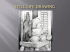### NIS-Elements: How to use Pixel Classifier to produce an Area Fraction... Pixel Classifier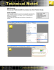### Orientate your home for healthy, comfortable living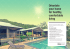### XMPlayer Express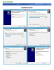### SolarVeil 2900 - Fraser Shading Systems Inc.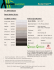### Pixel Perfect